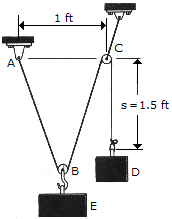# Engineering Mechanics - Equilibrium of a Particle - Discussion

### Discussion :: Equilibrium of a Particle - General Questions (Q.No.2)

2.A "scale" is constructed with a 4-ft-long cord and the 10-lb block D. The cord is fixed to a pin at A and passes over two small pulleys at B and C. Determine the weight of the suspended block E if the system is in equilibrium when s = 1.5 ft.

 [A]. W = 8.01 lb [B]. W = 14.91 lb [C]. W = 17.63 lb [D]. W = 18.33 lb

Explanation:

No answer description available for this question.

 Pratik/Satish said: (Aug 28, 2011) Considering traingle BCD between BC and CD is 23.57 degrees.so 10cos(23.57)= 9.165, double of which is the value of weight of block E i.e. 18.33.

 Ajinkya said: (Sep 28, 2011) Hey guys can you explain how did you get angle between bc and cd as 23.57 degrees ? please reply.

 Sidhu said: (Oct 4, 2011) Half of the distance of AC is 0.5 feet = BD and CD = 1.5 ft

 Mmwaris said: (Oct 6, 2011) Sidhu, let me explain you. its BC= 1.25ft (={4-1.5}/2), which will be used to calculate sin(theta) together with BD=0.5ft. i.e. Sin(theta) = 0.5/1.25=0.4 From here cos(theta) will be calculated. (theta is the angle at C ) Cos(theta)=sqrt{1-(sin(theta))square}=0.916 Then Tension in the rope T=M(D)*g -(1) And at E, the forces are M(E)*g=2*T*Cos(Theta) -(2) from (1) & (2) M(E)=2*M(D)*Cos(theta)=2*10*0.916=18.32 lb

 Srikanth said: (Oct 20, 2011) Can you please explain how does the tension in AB and BC became same i.e. T ?

 Raunak said: (Nov 19, 2011) Rope is of 4ft & ab=bc.... bc=(4-1.5)x1/2 =1.25 & by hypo... bd=0.5 now let angle at c=θ sin(θ)=0.5/1.25=0.4 cos(θ)=sqrt(1-sin2θ)=0.916 now, T,TENSION in rope T=MxDxg-------------(1) Forces at E, MxExg=2Tcosθ--------(2) from 1 & 2 we get MxE=2xMxDxcosθ =2x10x0.916 =18.32

 Nagu said: (Jun 14, 2012) Why we have to double the value ?

 Yuel said: (Aug 22, 2012) You can determine the weight of the suspended block 'E' BY USING COSINE LAW IN FORCE TRIANGLE. applying the law of cos in the triangle, we will obtain the ff: c = E a = 10 b = 10 ∝ = 132.84 where ∝ = 2θ - 180 ∝ = 2(23.58) - 180 ∝ = 132.84 substitute the value in cosine law where c^2 = a^2 + b^2 - 2abcos∝ E^2 = 10^2 + 10^2 - 2(10)(10)cos132.84 E = 18.33 XD

 Bala said: (Jul 22, 2013) @Sri kanth, I can explained them. AB=BC THAT STRUCTURE is triangle. Two side ninety degree in ago. A to C DISTANCE less than. Touch the the both way of two side return the value same.

 Ajeet Soni,Abbagoni Mahesh said: (Sep 29, 2015) Cos A = 0.5/1.25. Then a = 66.42. According to Lamis theorem We/sin (23.58+23.58) = 10/sin (90+66.42). We = 10xsin 47/sin 156 = 18.33.

 Prasanna said: (Oct 30, 2015) I didn't understand you are explanation.

 Ram said: (May 25, 2017) Anyone, can explain clearly?

 Balaji said: (Jun 9, 2018) (angle)BCD= 23.578. Then calculating the tension in string BC. Let it be called T. Now T is affected totally by the 10lb block. So, T= 10cos= 10cos23.578=9.1651. (Note : We're taking 10cos' because only the cos component of the force due to 10lb block is acting in string BC). Tension in AB = Tension in BC =T. Mass of E = T in AB +T in BC, So, 9.1651+9.1651= 18.3302 ~ 18.33lb.

 Siva said: (Aug 17, 2019) How do you find bc = 1.25?

 Kaliraj said: (Aug 12, 2020) I got the θ value as 18.34°. How all getting 23°? Please explain to me.